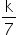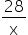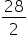Mathematics
Easy

Question

# Given that y varies inversely with x, if x = 7 and y = 4, what is y when x = 2 ?

## 14102-14Hint:

## The correct answer is: 14

### Here, x varies inversely to y.we have, y = k/x=>4 ==>k = 28.And, y === 14.Hence, the correct option is C.

In inverse variation x1y= x2y2.

### Related Questions to study#### With Turito Foundation.#### Get an Expert Advice From Turito.